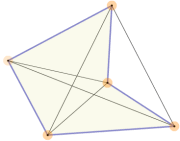# 多边形的对角线正方形有 2条对角线 八边形有 20条对角线

n边形的对角线个数是：

n(n − 3) / 2

### 例子：

• 正方形（或任何四边形）有 4(4−3)/2 = 4×1/2 = 2条对角线
• 八边形有 8(8−3)/2 = 8×5/2 = 20条对角线。
• 三角形有 3(3−3)/2 = 3×0/2 = 0条对角线。

### 自己来试试：对角线可以是在多边形外面的， 例如在一些凹多边形中。